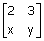# GATE | GATE CS 2010 | Question 29

Consider the following matrix
A =If the eigenvalues of A are 4 and 8, then

(A) x=4, y=10
(B) x=5, y=8
(C) x=-3, y=9
(D) x= -4, y=10

Explanation:Quiz of this Question

My Personal Notes arrow_drop_up
Article Tags :

Be the First to upvote.

Please write to us at contribute@geeksforgeeks.org to report any issue with the above content.